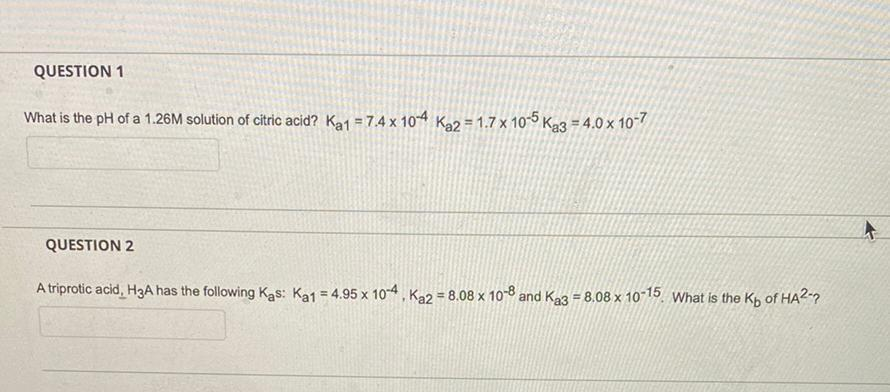Question:

# QUESTION 1 What is the pH of a 1.26M solution of citric acid? Ka1 = 7.4 x 10-4 Ka2 = 1.7 x 10-5 Ka3 = 4.0 x 10-7 QUESTION 2 A triprotic acid, H3A has the following Kas: Ka1 = 4.95 x 10-4, Ka2 = 8.08 xQUESTION 1 What is the pH of a 1.26M solution of citric acid? Ka1 = 7.4 x 10-4 Ka2 = 1.7 x 10-5 Ka3 = 4.0 x 10-7 QUESTION 2 A triprotic acid, H3A has the following Kas: Ka1 = 4.95 x 10-4, Ka2 = 8.08 x 10-8 and Ka3 = 8,08 x 10-15. What is the Kp of HA2-9